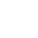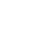LV. 49
GP 7k# 【心得】為什麼說捧蜂蜜與四葉(幸運)草是新世紀初最好看的戀愛「漫」畫(再看完動畫第二季)

GP4 BP-
0 何必提說剛看完原著漫畫三次?
^^^^^^^^^^^^^^^^^^^^^^^^^^^^^^^^^^^^^^^^^^^^^

1 粗糙的畫風表現細緻的心思
^^^^^^^^^^^^^^^^^^^^^^^^^^^^^^^^^^^

2 波希米亞跟森系風格的設定
^^^^^^^^^^^^^^^^^^^^^^^^^^^^^^^^^^^^^^

「天才」!

3 如一幅「畫」般詩意的展開
^^^^^^^^^^^^^^^^^^^^^^^^^^^^^^^^^^^^

4 漫畫以性愛做收尾的成熟感覺
^^^^^^^^^^^^^^^^^^^^^^^^^^^^^^^^^^^^^^^^^^

^^^^^^

LV. 50
GP 8kLV. 50
GP 8k3 樓 yau gx9900gundam
GP1 BP-

face基於日前微軟官方表示 Internet Explorer 不再支援新的網路標準，可能無法使用新的應用程式來呈現網站內容，在瀏覽器支援度及網站安全性的雙重考量下，為了讓巴友們有更好的使用體驗，巴哈姆特即將於 2019年9月2日 停止支援 Internet Explorer 瀏覽器的頁面呈現和功能。